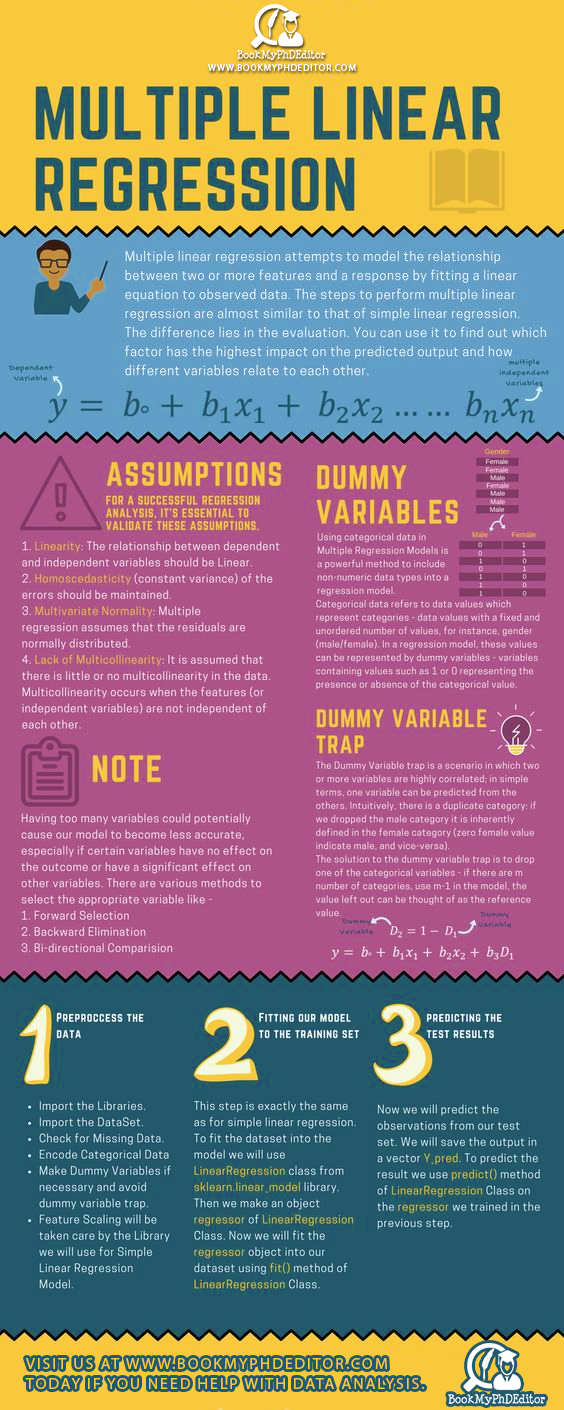Are you trying to understand the relationship between two or more independent variables and one continuous dependent variable? Do you intend to figure out which of the several explanatory variables has the highest impact on the predicted output and how different variables relate to each other? It is highly likely that you will find answers to these questions after you have deployed Multiple Linear Regression model to analyse the data set.

Take a look at the the above-given infographic to understand what is Multiple Linear Regression and what are the key assumptions to keep in mind when carrying out data analysis using this statistical technique.